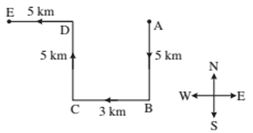# RRB ALP 2018 Practice Test Papers | Reasoning Questions (Day-5)

Dear Aspirants, Here we have given the Important RRB ALP & Technicians Exam 2018 Practice Test Papers. Candidates those who are preparing for RRB ALP 2018 can practice these Reasoning Questions to get more confidence to Crack RRB 2018 Examination.

[WpProQuiz 1480]

Click “Start Quiz” to attend these Questions and view Solutions

1. In the following sequence of alphabets a a b a b a a b a b a aa b b a b a b b a aaa the number of a’s in between 7th a from left and 7th a from right is—

(A) 1

(B) 0

(C) 3

(D) 2

1. Game: Field:: Cinema:?

(A) Hall

(B) Stage

(C) Screen

(D) Drama

1. Which one of the pairs is different from the rest of the four pairs?

(A) Society—Member

(B) Office—Worker

(C) Government—Public

(D) Team—Player

1. In the question given below five words are given out of which four are similar in a certain way while the rest one is different. Find out the different one.

(A) CGNX

(B) IMTD

(C) JNUE

(D) ORYJ

1. Find out the number that would replace the question mark (?) in each of the following questions— 20, 32, 45, 59, 74,?

(A) 95

(B) 90

(C) 85

(D) 79

1. If ‘1 + 2 + 3’ means ‘some are brave’. ‘2 + 3 + 4’ means ‘some are cowards’ and ‘3 + 4 + 5 + 6’ means ‘some cowards have come’; which digit means ‘are’ in that language?

(A) 1

(B) 3

(C) 2

(D) 4

1. Mohit walks 5 km to South, then he walks 3 km turning to his right. Again he turns to his right and walks 5 km. After this turning to his left he walks 5 km. In which direction and how far is he now from the starting point?

(A) 5 km, West

(B) 3 km, North

(C) 3 km, East

(D) 8 km, West

1. If by looking in a mirror it appears that it is 9: 30 in the clock, what is the real time?

(A) 4: 30

(B) 6: 30

(C) 2: 30

(D) 6: 10

1. If ‘A + B’ means A is the brother of B, ‘A – B’ means A is the sister of B, ‘A × B’ means A is mother of B and ‘A ÷ B’ mean A is the father of B, which of the following means P is the maternal uncle of Q?

(A) P × Q – S

(B) P – S × Q

(C) P + S × Q

(D) P – Q × S

1. If the 1st and 6th letters of the word ‘PHOTOGRAPH’ are interchanged, also 2nd and 7th letters, and so on, which of the following would be the 4th letter from your right?

(A) H

(B) P

(C) O

(D) T

Explanation:

There is only one ‘a’ between 7th a from left and 7th a from right.

As the place of game is field similarly the place of cinema is ‘Hall’.

In all the rest pairs, second word is the part of first.

In all the rest terms three letters according to alphabet are missing between first and second letters, six letters are missing between second and third letters and nine letters are missing between third and fourth letters.

The differences of two consecutive numbers are 12, 13, 14, 15 and 16 respectively.

By comparing first, second and third we get 3 is used for ‘some’ because 3 and some are common. Now on comparing first and second we get 2 + 3 is used for ‘some are’. Therefore, it is clear that for ‘are’ ‘2’ is used. Hence, the correct answer is (C).Mohit is at a distance of 8 km from his starting point in West direction.

When the hour hand of the clock is between 2 and 3, it will appear to between 9 and 10 in the mirror and when the minute hand is on 6 it will appear in the mirror on 6. Thus when it is actually 2: 30 in a clock it will appear 9: 30 in the mirror.

P + S × Q means P is the brother of S and S is the mother of Q. Therefore, P is the maternal uncle of Q.

After interchanging the letters according the instruction the word ‘GRAPHPHOTO’ is formed. Its 4th letter from the right is H.

RRB ALP 2018 Practice Test Papers | Reasoning Questions (Day-1)

RRB ALP 2018 Practice Test Papers | Reasoning Questions (Day-2)

RRB ALP 2018 Practice Test Papers | Reasoning Questions (Day-3)

RRB ALP 2018 Practice Test Papers | Reasoning Questions (Day-4)